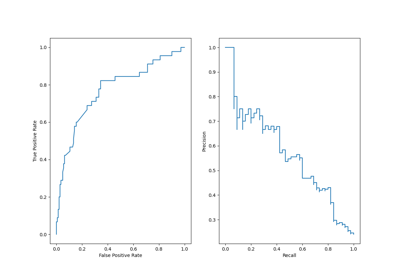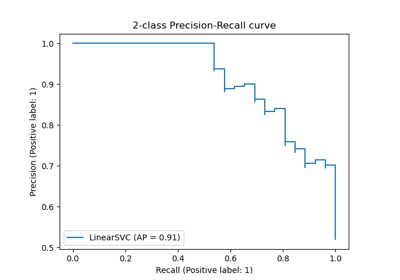# sklearn.metrics.precision_recall_curve¶

sklearn.metrics.precision_recall_curve(y_true, probas_pred, *, pos_label=None, sample_weight=None)[source]

Compute precision-recall pairs for different probability thresholds.

Note: this implementation is restricted to the binary classification task.

The precision is the ratio tp / (tp + fp) where tp is the number of true positives and fp the number of false positives. The precision is intuitively the ability of the classifier not to label as positive a sample that is negative.

The recall is the ratio tp / (tp + fn) where tp is the number of true positives and fn the number of false negatives. The recall is intuitively the ability of the classifier to find all the positive samples.

The last precision and recall values are 1. and 0. respectively and do not have a corresponding threshold. This ensures that the graph starts on the y axis.

The first precision and recall values are precision=class balance and recall=1.0 which corresponds to a classifier that always predicts the positive class.

Read more in the User Guide.

Parameters:
y_truendarray of shape (n_samples,)

True binary labels. If labels are not either {-1, 1} or {0, 1}, then pos_label should be explicitly given.

probas_predndarray of shape (n_samples,)

Target scores, can either be probability estimates of the positive class, or non-thresholded measure of decisions (as returned by decision_function on some classifiers).

pos_labelint or str, default=None

The label of the positive class. When pos_label=None, if y_true is in {-1, 1} or {0, 1}, pos_label is set to 1, otherwise an error will be raised.

sample_weightarray-like of shape (n_samples,), default=None

Sample weights.

Returns:
precisionndarray of shape (n_thresholds + 1,)

Precision values such that element i is the precision of predictions with score >= thresholds[i] and the last element is 1.

recallndarray of shape (n_thresholds + 1,)

Decreasing recall values such that element i is the recall of predictions with score >= thresholds[i] and the last element is 0.

thresholdsndarray of shape (n_thresholds,)

Increasing thresholds on the decision function used to compute precision and recall where n_thresholds = len(np.unique(probas_pred)).

PrecisionRecallDisplay.from_estimator

Plot Precision Recall Curve given a binary classifier.

PrecisionRecallDisplay.from_predictions

Plot Precision Recall Curve using predictions from a binary classifier.

average_precision_score

Compute average precision from prediction scores.

det_curve

Compute error rates for different probability thresholds.

roc_curve

Compute Receiver operating characteristic (ROC) curve.

Examples

>>> import numpy as np
>>> from sklearn.metrics import precision_recall_curve
>>> y_true = np.array([0, 0, 1, 1])
>>> y_scores = np.array([0.1, 0.4, 0.35, 0.8])
>>> precision, recall, thresholds = precision_recall_curve(
...     y_true, y_scores)
>>> precision
array([0.5       , 0.66666667, 0.5       , 1.        , 1.        ])
>>> recall
array([1. , 1. , 0.5, 0.5, 0. ])
>>> thresholds
array([0.1 , 0.35, 0.4 , 0.8 ])


## Examples using sklearn.metrics.precision_recall_curve¶Visualizations with Display Objects

Visualizations with Display ObjectsPrecision-Recall

Precision-Recall